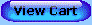For a lifetime of learning fun!

If you are unable to see the blue navigation buttons below, go to Contents for text links.1. Fundamental Operations
2. Functions and Graphs
3. The Integers
4. The Rational Numbers
5. Equations in One Variable
6. Equations in Two Variables
7. Simultaneous Equations
8. Exponents

MIDTERM REVIEW

9. Polynomials
10. Factoring
11. Fractions
12. Square Roots